# Measuring Pressure

Pressure is a very important component of a fluid mechanics.  Due this fact having a way to measure pressure is very important.  It is so important that multiple devices and techniques are used to measure pressure. You first need to decide if you are interested in measuring gage pressure or absolute pressure.  Recall that absolute pressure measures the total pressure in an environment.  This includes atmospheric pressure as well as gage pressure.  Gage pressure on the other hand only measures the change in pressure of an object.  It does not consider atmospheric pressure.  An atmospheric pressure is always positive while gage pressure can be both positive and negative.

### Measuring Atmospheric Pressure

To measure atmospheric pressure you would use a barometer.  A simple barometer consists of a glass tube. One end of the glass tube is closed and the other end is submerged in a bath of mercury.  To set up a barometer the tube is first filled with mercury. Once you fill the tube with mercury you will place it upside down into a container of mercury.  A vapor pressure will develop inside the open space inside the tube that will counteract the weight of the mercury keeping it in the tube.  Once the barometer is setup the level of the mercury within it will change as the atmospheric pressure changes.

(Eq 1) $p_{atm} = γh + p_{vapor}$

$γ$ = Specific Weight

Note other liquids can be used.  However, mercury is the most practical due to its large specific weight in comparison to other liquids.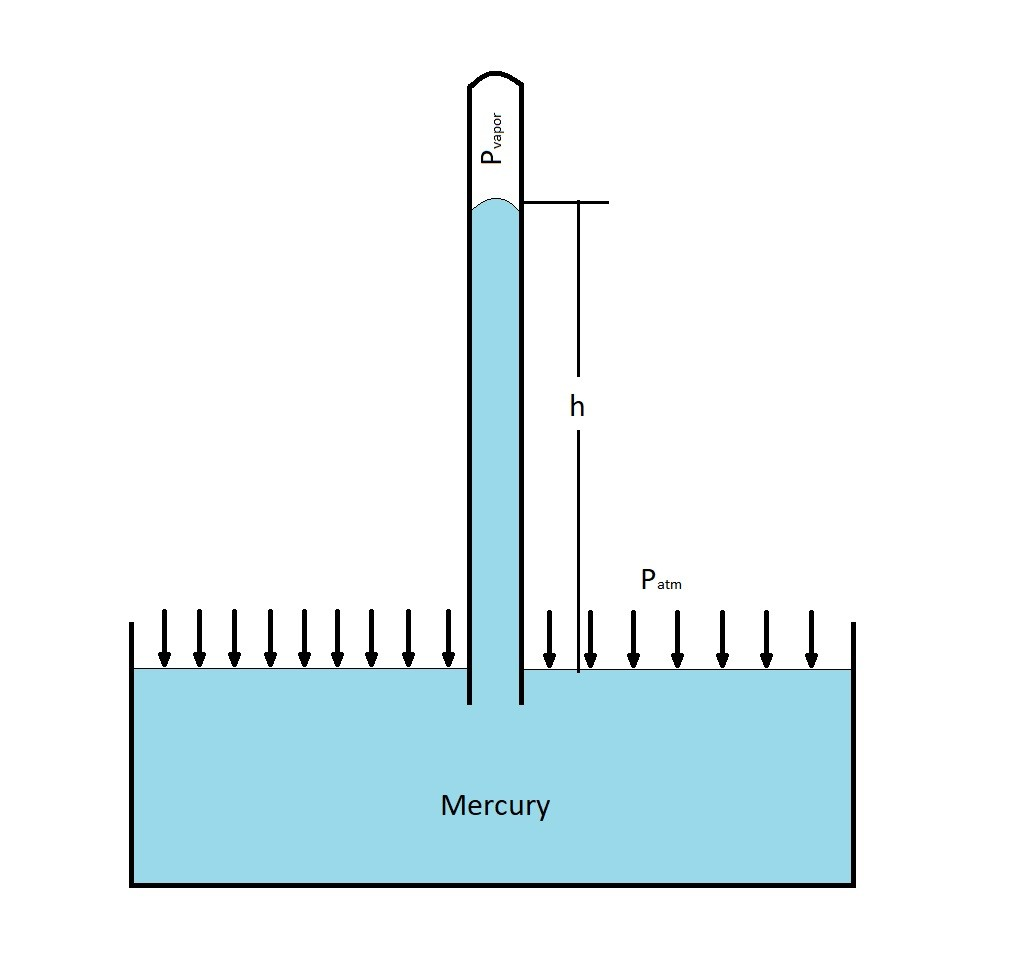### Manometers

Another method that is used for measuring pressure is the use of manometers.  A manometer uses vertical or inclined tubes that are filled with a liquid to measure pressure.  As the pressure changes the level of the liquid inside of the tube will change.

##### Piezometer Tube

One of the simplistic types of manometers is called the piezometer tube.  The piezometer tube is a simple vertical tube that is open to atmosphere on the top.  It is than attached to  a container to measure the pressure of that container. to find the absolute pressure of the container you would use the following equation.

(Eq 2) $p=γh+p_o$

$p_o$ = atmospheric pressure

On the other hand, if you are only interested in the gage pressure, then you would only need to use the first part of equation 2.

(Eq 3) $P_A=γ_1h_1$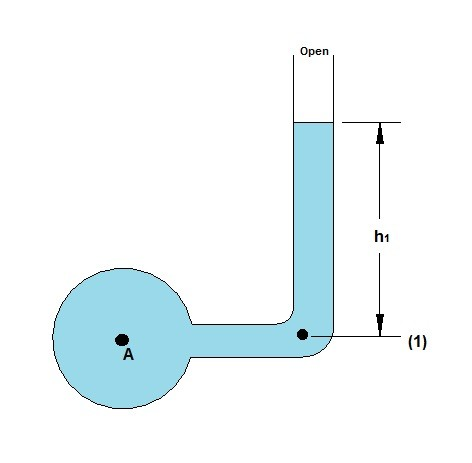As the fluid moves upward there will be an increase in pressure.  In contrast if the fluid were to move down there is a decrease in pressure.

The piezometer is a very simple and accurate measuring device. It does however have several disadvantages.  First the pressure inside the container must be greater than atmosphere.  Otherwise air will be sucked into the system.  Next, changes in pressure must be relatively small so that the height of the tube will be reasonable.  Finally, the fluid in the container can only be liquid.  Meaning this device cannot measure the pressure change in a gas.

##### U-Tube Manometer

Due to the issues noted above for the piezometer manometer the u-tube manometer was invented.  The U-tube manometer was designed to have a U-bend in its design that holds a separate fluid called the gage fluid.  This will allow the manometer to measure the pressure of both gas and liquid, since the gage fluid will act as barrier to the fluid in the reservoir.  You can have two different types of u-tube manometers.  One that has an end open to atmosphere.  The other type will have reservoir connected to both ends.

First let’s discuss the u-tube manometer that is open to atmosphere.  To determine the gage pressure within the reservoir you would use the following equation.

(Eq 4) $P_A=γ_2h_2-γ_1h_1$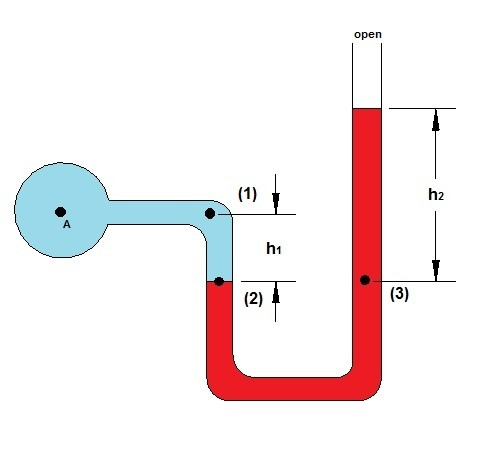The next type of u-tube manometer is the type that connects two different reservoirs.  By doing this you will be able to see what the pressure difference is between the two reservoirs.  A real life example of when this would be used is when you want to measure the pressure drop of a plumbing system between to critical points in the system.  To determine the pressure change the following equation would be used.

(Eq 5)  $P_A-P_B=γ_2h_2+γ_3h_3-γ_1h_1$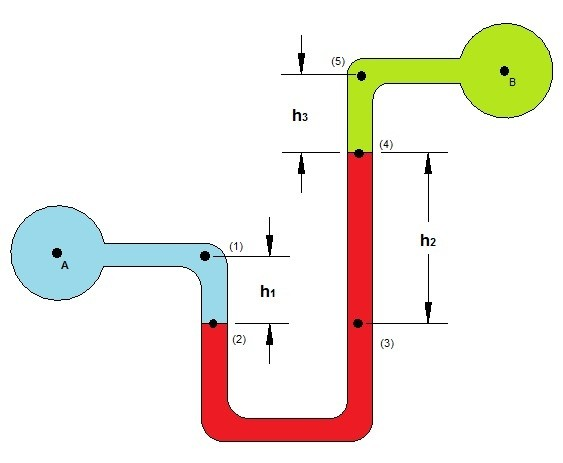##### Incline Tube Manometer

Finally, a third type of manometer is called the incline manometer.  The incline manometer is used when you want to increase the accuracy of your pressure measurement.  This is done by using a tube filled with a gage fluid that is set an incline instead of vertically.  By setting the tube at an incline the change in fluid level will be more pronounced.  However, by doing this you will decrease the range of pressure change that the manometer will be able to measure.  Below is an example of an incline tube manometer that is measuring the pressure change between two different reservoirs.  However, you can also have an incline tube manometer that is open to atmosphere at one end.

(Eq 6) $P_A-P_B=γ_2l_2sinθ+γ_3h_3-γ_1h_1$

γ = Specific Weight

P = Pressure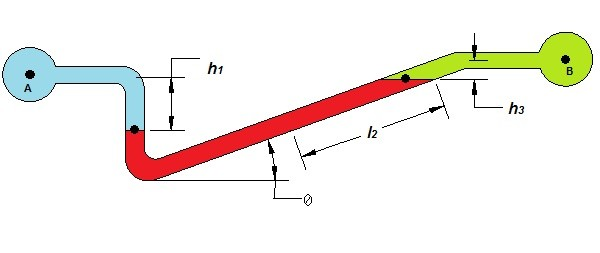### Other Measuring Devices

Manometers are a popular device to use to measure pressure.  They are however are not the best device to use to measure vacuum pressure, very high pressures, or pressures that change rapidly with time.  To overcome these problems other devices for measuring pressure have been invented.  Most of these devices rely on an elastic structure that deform.  The amount the structure deforms the greater the pressure change.

An example of a pressure gage that requires an elastic structure to deform is a Bourdon pressure gage.  A Bourdon pressure gage consists of a Boardon tube, a link connecting the tube to gear with a hair spring.  As the pressure inside the Bourdon tube changes it will flex which will in turn move the point on the pointer giving you a pressure measurement.  These type of gauges can be made to measure both positive and negative gauge pressure.  However, zero pressure when the gauge is calibrating correctly always represents atmospheric pressure.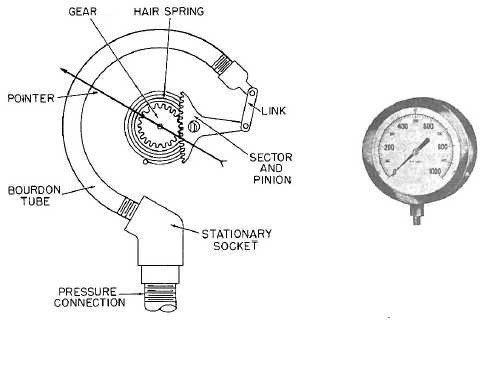Another type of mechanical gage is the aneroid barometer.  The aneroid barometer unlke the Bourdon gage is used to measure atmospheric pressure.  This is done by evacuating a hollow area within the gauge making it almost a perfect vacuum.  A thin membrane is than placed over this evacuated area that will flex when the atmospheric pressure changes.

Finally, linear variable differential transformers (LVDT) can be used to measure pressure.  These devices work by measuring a voltage change in the LVDT due to the movement of a mechanical device, such as a Bourdon Tube or diaphragm.  The voltage change is than converted into a pressure change.  The advantage of using an LVDT is the data can be digitized and stored for later processing on a computer.

|